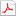검색어 입력폼

# [전자기학] 전자기학 McGrawHill 연습문제풀이7장

등록일 2002.10.30어도비 PDF (pdf) | 9페이지 | 가격 3,000원

## 목차

7.1.Let V =2 xy 2 z 3 and =.Given point P (1 ,2 , 1),ﬁnd:

7.3.Let V (x,y )=4 2 x +f (x ) −3 y 2 in a region of free space where ┭ v =0.It is known that both
E and V are zero at the origin.Find f (x )and V (x,y ):

7.5.Given the potential ﬁeld V =(A┭ 4 +B┭ −4 )sin 4 ┵ :

7.7.Let V =(cos 2 ┵ )/┭ in free space.
a)Find the volume charge density at point A (0 .5 ,60 ◦,1):Use Poisson ’s equation:

7.9.The functions V 1 (┭,┵,z )and V 2 (┭,┵,z )both satisfy Laplace ’s equation in the region a <┭ <b ,
0 ●┵<2 ┪ , −L <z<L ;each is zero on the surfaces ┭ =b for −L <z<L ;z = −L for
a <┭ <b ;and z =L for a <┭ <b ;and each is 100 Von the surface ┭ =a for −L <z<L .

7.11.The conducting planes 2 x +3 y =12 and 2 x +3 y =18 are at potentials of 100 Vand 0,
respectively.Let =0 and ﬁnd:
...

## 본문내용

7.9.The functions V 1 (┭,┵,z )and V 2 (┭,┵,z )both satisfy Laplace ’s equation in the region a <┭ <b ,
0 ●┵<2 ┪ , −L <z<L ;each is zero on the surfaces ┭ =b for −L <z<L ;z = −L for
a <┭ <b ;and z =L for a <┭ <b ;and each is 100 Von the surface ┭ =a for −L <z<L .
a)In the region speci ﬁed above,is Laplace ’s equation satis ﬁed by the functions V 1 +V 2 ,
V 1
−V 2 ,V 1 +3,and V 1 V 2 ?Yesfor the ﬁrst three,since Laplace ’s equation is linear.No
for V 1 V 2 .
b)On the boundary surfaces speci ﬁed,are the potential values given above obtained from
the functions V 1 +V 2 ,V 1
−V 2 ,V 1 +3,and V 1 V 2 ?At the 100 Vsurface (┭ =a ),No for all.
At the 0 Vsurfaces,yes,except for V 1 +3.
c)Are the functions V 1 +V 2 ,V 1
−V 2 ,V 1 +3,and V 1 V 2 identical with V 1 ?Only V 2 is,
since it is given as satisfying all the boundary conditions that V 1 does.Therefore,by the
uniqueness theorem,V 2 =V 1 .The others,not satisfying the boundary conditions,are
not the same as V 1 .

## 참고 자료

McGrawHill 연습문제풀이7장